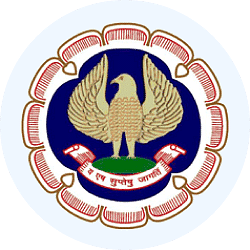MCQ - Statistical Description of Data

# MCQ - Statistical Description of Data - Business Mathematics and Logical Reasoning & Statistics - CA Foundation

``` Page 1

CPT  Section D Quantitative Aptitude
Chapter 10
Dr. R. B. Tiwari
Page 2

CPT  Section D Quantitative Aptitude
Chapter 10
Dr. R. B. Tiwari
Question Time
Page 3

CPT  Section D Quantitative Aptitude
Chapter 10
Dr. R. B. Tiwari
Question Time
a) Economics
c) Commerce and Industry
d) Always
Page 4

CPT  Section D Quantitative Aptitude
Chapter 10
Dr. R. B. Tiwari
Question Time
a) Economics
c) Commerce and Industry
d) Always
(a) A qualitative characteristics
(b) A Quantitative Characteristics
(c) A Measurable Characteristics
(d) All These
Page 5

CPT  Section D Quantitative Aptitude
Chapter 10
Dr. R. B. Tiwari
Question Time
a) Economics
c) Commerce and Industry
d) Always
(a) A qualitative characteristics
(b) A Quantitative Characteristics
(c) A Measurable Characteristics
(d) All These
(a) An attribute
(b) A discrete variable
(c) A continuous variable
(d) (b)  or (c)
```

## Business Mathematics and Logical Reasoning & Statistics

128 videos|128 docs|87 tests

## FAQs on MCQ - Statistical Description of Data - Business Mathematics and Logical Reasoning & Statistics - CA Foundation

 1. What is statistical description of data?Ans. Statistical description of data refers to the process of summarizing and analyzing data using statistical methods. It involves organizing and presenting data in a meaningful way to understand its characteristics, patterns, and trends.
 2. Why is statistical description of data important?Ans. Statistical description of data is important because it helps in making informed decisions based on data analysis. It provides insights into the data, helps in identifying patterns and trends, and allows for comparisons between different groups or variables. It also helps in understanding the variability and distribution of data.
 3. What are the different measures of central tendency used in statistical description of data?Ans. The measures of central tendency used in statistical description of data are: - Mean: It is the average value and is calculated by summing up all the values and dividing it by the total number of values. - Median: It is the middle value of a data set when arranged in ascending or descending order. It divides the data into two equal halves. - Mode: It is the value that occurs most frequently in a data set.
 4. How are measures of dispersion useful in statistical description of data?Ans. Measures of dispersion are useful in statistical description of data as they provide information about the spread or variability of the data. They help in understanding how the data points are distributed around the measures of central tendency. Some commonly used measures of dispersion are range, variance, and standard deviation.
 5. What is the difference between descriptive statistics and inferential statistics in statistical description of data?Ans. Descriptive statistics involves summarizing and describing data using measures such as mean, median, mode, and measures of dispersion. It focuses on describing the characteristics of the data set. In contrast, inferential statistics involves making inferences or drawing conclusions about a population based on a sample. It uses probability theory and hypothesis testing to make predictions or generalizations about the population using sample data.

## Business Mathematics and Logical Reasoning & Statistics

128 videos|128 docs|87 testsExplore Courses for CA Foundation examSignup to see your scores go up within 7 days! Learn & Practice with 1000+ FREE Notes, Videos & Tests.
10M+ students study on EduRev
Track your progress, build streaks, highlight & save important lessons and more!
Related Searches

,

,

,

,

,

,

,

,

,

,

,

,

,

,

,

,

,

,

,

,

,

;